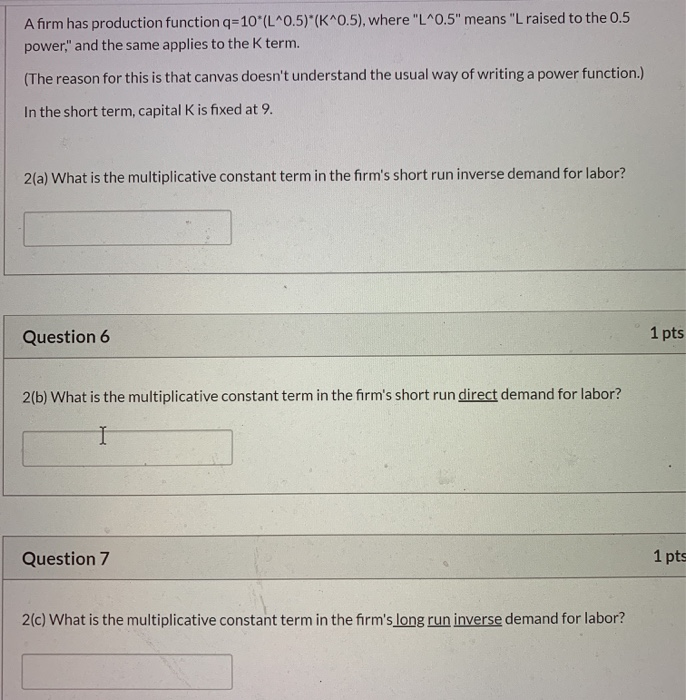### Create an Account

Already have account?

### Forgot Your Password ?

Home / Questions / Afirm has production function q 10L^0.5)*K^0.5, where L05 means L raised to the 0.5

# Afirm has production function q 10L^0.5)*K^0.5, where L05 means L raised to the 0.5Afirm has production function q=10"(L^0.5)*(K^0.5), where "L"0.5" means "L raised to the 0.5 power," and the same applies to the K term. (The reason for this is that canvas doesn&#39;t understand the usual way of writing a power function.) In the short term, capital K is fixed at 9. 2(a) What is the multiplicative constant term in the firm&#39;s short run inverse demand for labor? Question 6 1 pts 2(b) What is the multiplicative constant term in the firm&#39;s short run direct demand for labor? Question 7 1 pts 2(c) What is the multiplicative constant term in the firm&#39;s long run inverse demand for labor?

Feb 07 2020 View more View Less

#### Answer (UnSolved)Get Solution